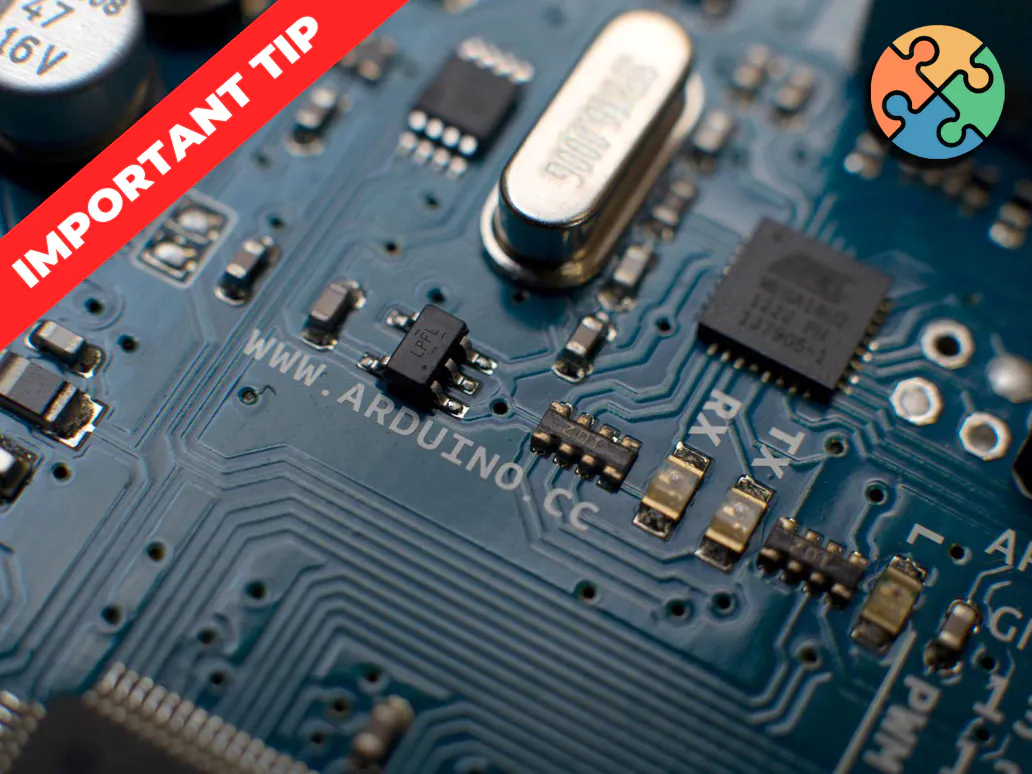Project tutorial# Increase the Electrical Current Capacity of the Arduino Pins © GPL3+

Learn how to create a circuit to increase the electrical current of the Arduino pins to activate loads that need more electrical current.

• 4,732 views
• 30 respects

## Apps and online services

### Introduction

Between the several types of Arduino and other Microcontrollers, we have a problem: the low capacity of electrical current sourcing of the pins. Where this value can vary between 6 mA to 40 mA in some models. But be careful, always check the datasheet of your device and check the electrical current sourcing capacity of the pins.

But now, to solve this problem, we will use an electrical current driver circuit and we will present all step by step to you construct your own circuit of simple way.

### Project Development

Through the presented problem, the circuit allows you to drive a load whose electrical current is higher than the pin of the Microcontroller can provide. And for that, we will use a transistor.

The transistor will be used as a switch and will allow greater electrical current capacity for the load. And for this, we will use this circuit, that is presented in Figure 2, connected to the Arduino pin or any other CHIP. This circuit can be replicated to all digital pins.

As is possible analyze, in this the circuit we use a transistor connected at the Arduino pin through a resistor (R1).

Now, will be presented the process to design all circuit through three steps:

First, we must calculate the base current, which is 10% of the load current value. This electric current will be supplied by the Arduino pin. For this, we will consider that the load requires 100 mA, our value of Ib (electrical current to active the transistor base) will be 10 mA.

For each type of load, you need to know what is the electrical current value to drive the load.

Now, note that with a low electric current at the base of the Transistor, we trigger a load that requires a current value 10 times higher.

Therefore, with the value of the base current, we go to the second step: Calculate the resistance of the base with this equation.

``````Rb = (VDD - 0.7)/Ib                     -                      Equation (1)
``````

Substituting 10 mA into Ib, we find the resistor value of 430R, which is a commercial value.

In the third step, we will dimension the transistor. For this solution, we use NPN transistor BC337 because it supports a collector electrical current of 800 mA, which is larger than the load current.

And the load will be connected in the terminal +load and -load, as is shown in Figure 1.

Therefore, through this project, is possible increase the electrical current sourcing capacity of arduino pins and create projects with loads fo more power.

### Our Last Published Article

Learn how to create the MyPet Maker Board and earn 10 free Printed Circuit Boards. Access this link!

### Acknowledgments

The Silícios Lab thanks PCBWay for its support and work together.

In addition to them, the Silícios Lab thanks UsinaInfo Store for its support, for offering us the electronic components of great quality and good service.

And Ubidots, for offering us its excellent platform of Internet of Things, for the development of new projects.

## Schematics#### Standalone Arduino Applied in Projects

Project tutorial by PCBWay and Silícios Lab

• 7,265 views
• 47 respects

#### Security Battery for Arduino and Electronic Devices

by Silícios Lab and PCBWay

• 4,842 views
• 22 respects

#### Creating a Datalogger with Arduino - Part I

Project tutorial by PCBWay and Silícios Lab

• 1,444 views
• 6 respects

#### Storing the Charge State with Arduino

by PCBWay and Silícios Lab

• 527 views
• 4 respects

#### Smart BT Door Lock (Simple Arduino)

Project showcase by Arun

• 10,828 views
• 1 comment
• 22 respects

#### Arduino Uno + Electrical Device (Bulb) + Android Phone

Project tutorial by Stephen Simon

• 9,183 views